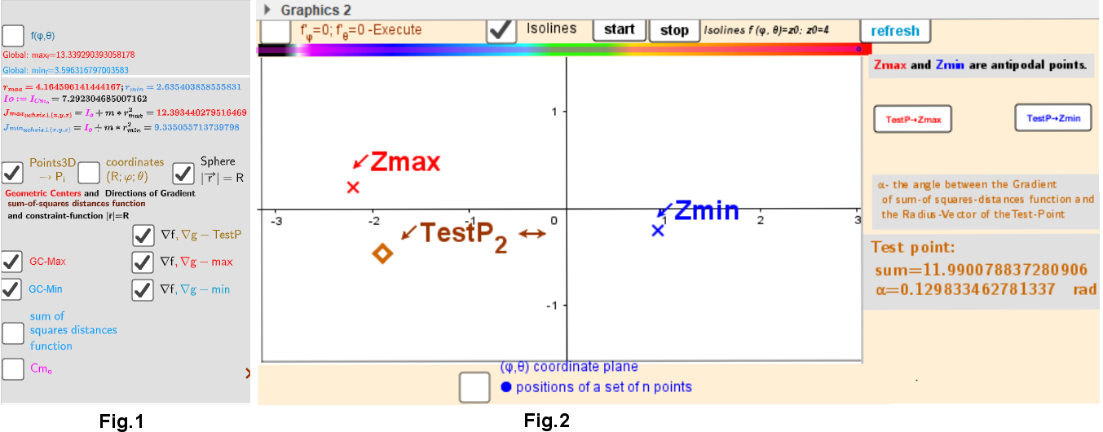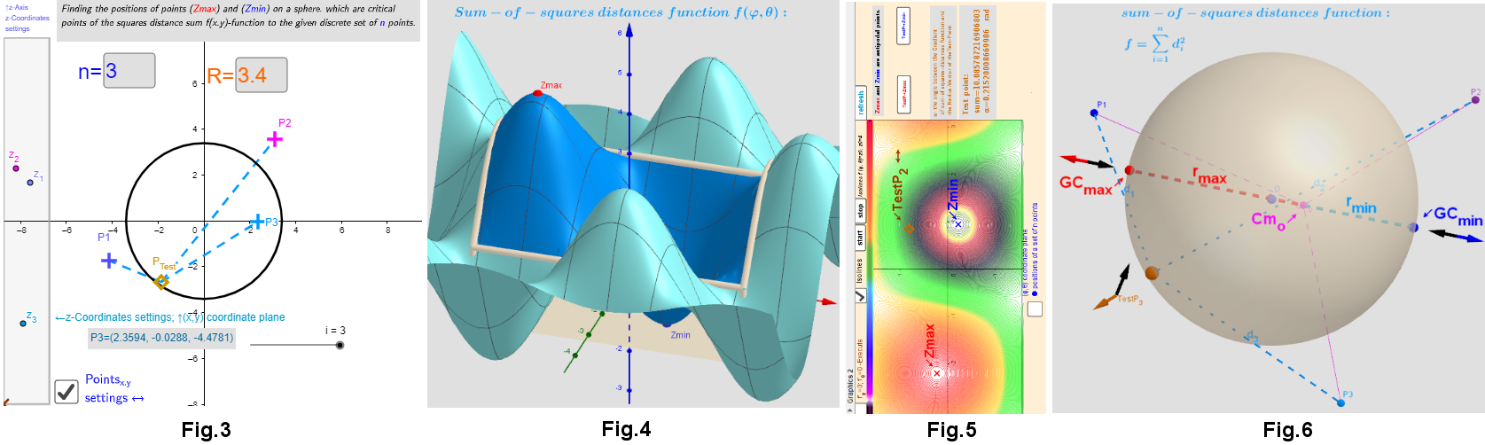# Description. Geometric Centers on a Sphere.

Previously, we considered the case of finding geometric centers on a circle generated by a system of points that are somehow distributed in space. Description. Finding Geometric Medians and Geometric Centers on a circle from discrete sample points. Applet. Finding Geometric Medians and Geometric Centers on a circle from discrete sample points. Finding the location of geometric medians/centers on the circle from discrete sample points depending on the position of the test point. Applet is used to study the distribution of geometric centers on a sphere of radius R, „induces“ by the discrete sample of movable points in the 3-D space. There is a set lP={P1, P2,...,Pn} of points and {(xi,yi,zi)∈ℝ3:i = 1,...,n} -their coordinates. Coordinates (xi,yi) are set by moving the Pi points and the coordinates zi -by moving the zi-points (Fig. 3). The point Cm minimizes a function of the sum of squared distances and is called the center of mass https://en.wikipedia.org/wiki/Center_of_mass, centroid or Geometric Center https://en.wikipedia.org/wiki/Centroid of the set lP. Problem: find the positions of critical points (global minima and maxima too) of the function of squares of the sum of distances not only in the entire in the 3-D space, but on its bounded area. Definitions of geometric centers can be generalized, in the sense that the critical points of the squares of the sum of distances function searched on a bounded area of 3-D space.

## Geometric Centers on a bounded area (on a sphere), „induced“ by the points from 3-D space

The Geometric Center is defined here as point on sphere from where the sum of the squares of all Euclidean distances to each point pi's have at that point: local minimum, maximum or a saddle points. Critical points can be found using Lagrange multipliersas (Λ(x,y,z,λ)=f(x,y,z)+λ*g(x,y,z)) finding the Extreme values of the function : fq(x,y,z)= -sum of the squares of the distances to the points pi, subject to a constraining equation: g(x,y,z)=x2+y2+z2-R2 - points (x,y,z)∈S. I.e. it is necessary to find the critical points fq(x,y,z) subject to: g(x,y,z)=0. Let's denote the resulting point as Sum(lP):= , then ∇f/2=n*(x,y,z)- or ∇f/2=n*(x,y,z)-Sum(lP) and ∇g/2=(x,y,z): ⇒(x,y,z)∼Sum(lP). The point (x,y,z) (Fig.6) that minimize the sum of the squares of the distances to the points pi is the Geometric Center (gravity center, barycenter, center of mass, centroid) GC: Cm= -its coordinates are the averages of the coordinates of the points from set lP. https://en.wikipedia.org/wiki/Center_of_mass .☛ In this problem there are only two critical points (x,y,z)∈S. The Axis passing through the radius vector of the point Sum(lP) passes through the center of mass Cm and intersects the sphere S at two points: one corresponds to the global maximum, the other- to the global minimum (Fig.6). Their coordinates have explicit formulas: GCmin= R* UnitVector(Sum(lP)) and GCmax= - R*UnitVector(Sum(lP)) - two antipodal points on sphere. These points can be found using the Steiner theorem too (Fig.1, rmax and rmin are shown in the Fig.6). f(x,y,z): In the spherical coordinate system we will have a two-variable function f(φ,θ) over a rectangular region: - π ≤φ≤ π and π/2≤θ≤π/2. The two-dimensional surface plot of f(φ,θ) -function of sum of squares of the distances relative to the relative to the Test point with angular position (φ,θ) on a sphere of radius R for a given location of points from the set lP is shown in Fig. 4(Checkbox-"f(φ,θ)"=true). The solution (critical points of a function f(x,y,z)) of the system of equations can be found as intersection points of the corresponding implicit functions f'φ=0; f'θ=0, as shown in Fig. 2, or using isolines: Fig.5. The test point is set by moving the "TestP2↔" point in the plane (φ, θ): Fig.2.Distribution of points from lP in 3D and corresponding 2 critical points GCmin∈S and GCmax∈S, directions for them from "resulting of all vectors: ∼Sum(lP)" and position vectors on the surface of a sphere is shown in Fig. 6(Checkbox-"Points3D"=true). For illustration purposes, the vectors are attached to the points. With applet you can visually observed and explore 2 geometric centers: GCmin and GCmax on a sphere S , geometric center Cm in ℝ3 (Fig. 6) changing the positions of the points from lP.